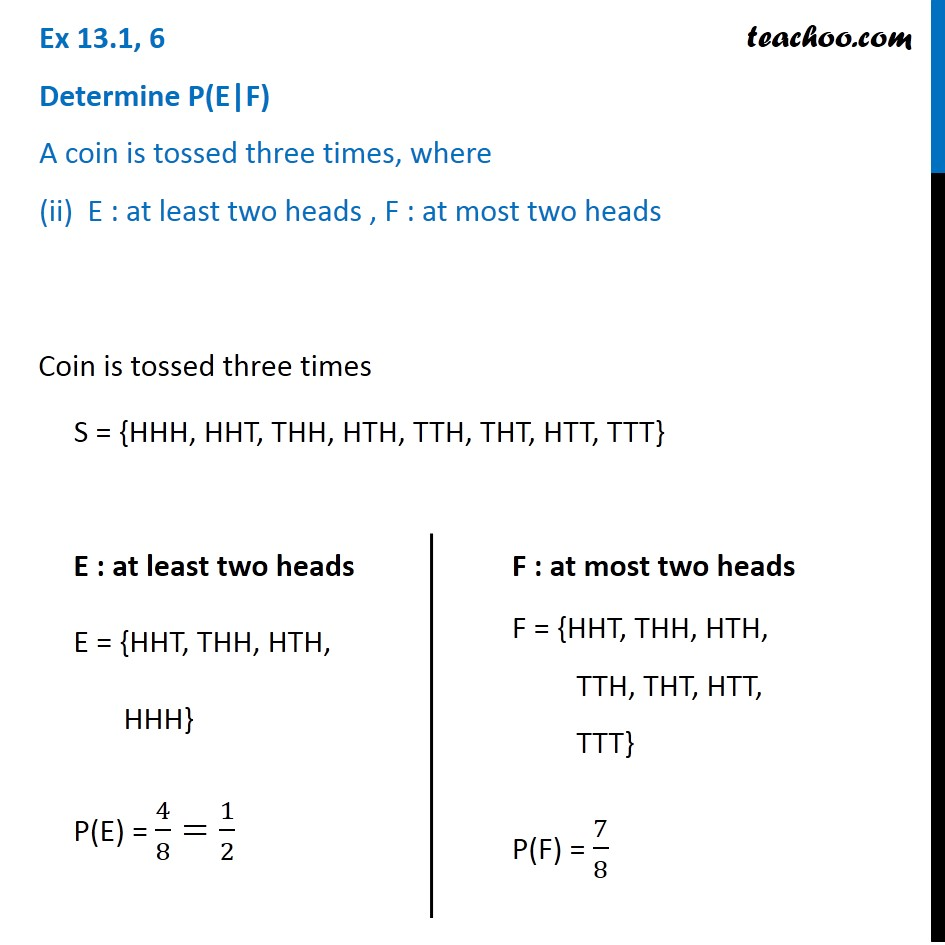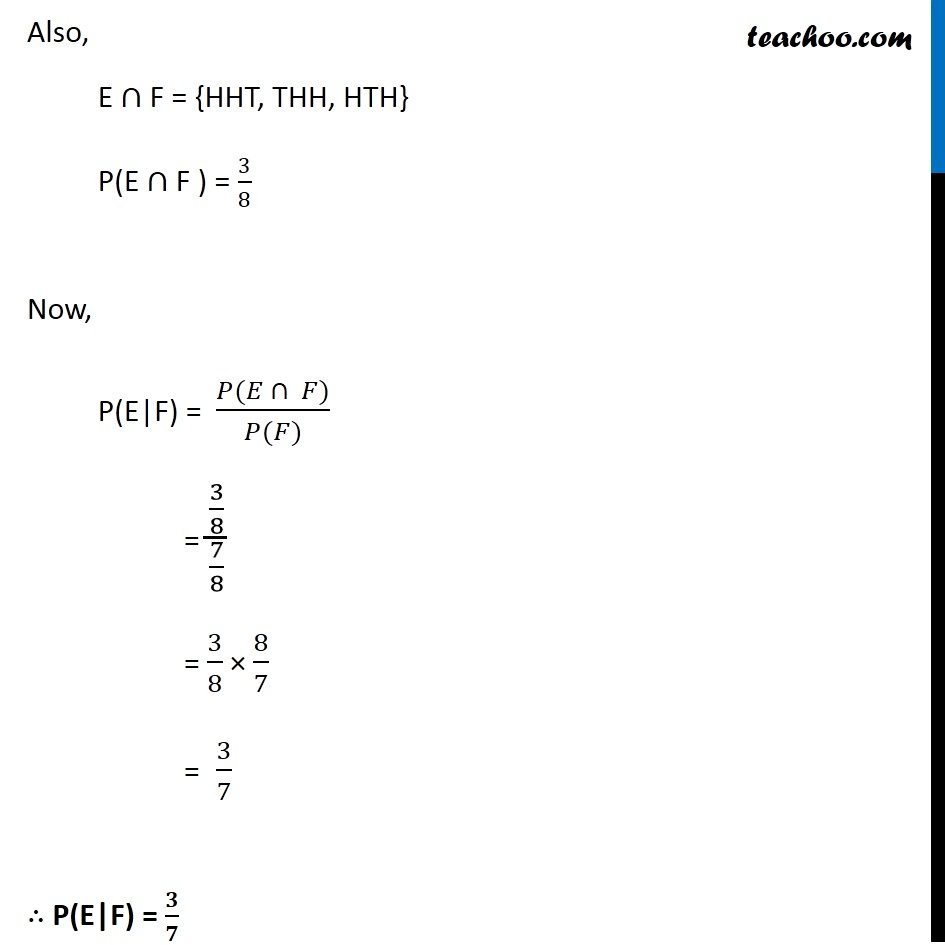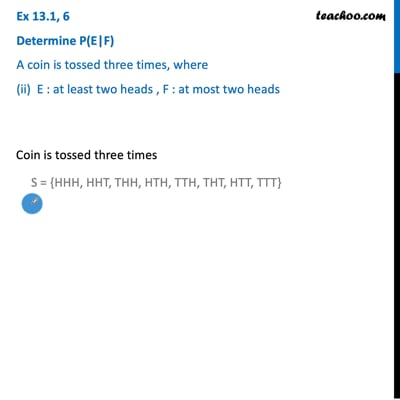Ex 13.1

Chapter 13 Class 12 Probability
Serial order wiseThis video is only available for Teachoo black users

Introducing your new favourite teacher - Teachoo Black, at only ₹83 per month

### Transcript

Ex 13.1, 6 ﻿Determine P(E|F) A coin is tossed three times, where (ii) E : at least two heads , F : at most two heads Coin is tossed three times S = {HHH, HHT, THH, HTH, TTH, THT, HTT, TTT} Coin is tossed three times S = {HHH, HHT, THH, HTH, TTH, THT, HTT, TTT} E : at least two heads E = {HHT, THH, HTH, HHH} P(E) = 4/8=1/2 F : at most two heads F = {HHT, THH, HTH, TTH, THT, HTT, TTT} P(F) = 7/8 Also, E ∩ F = {HHT, THH, HTH} P(E ∩ F ) = 3/8 Now, P(E|F) = (𝑃(𝐸 ∩ 𝐹))/(𝑃(𝐹)) = (3/8)/(7/8) = 3/8 × 8/7 = 3/7 ∴ P(E|F) = 𝟑/𝟕